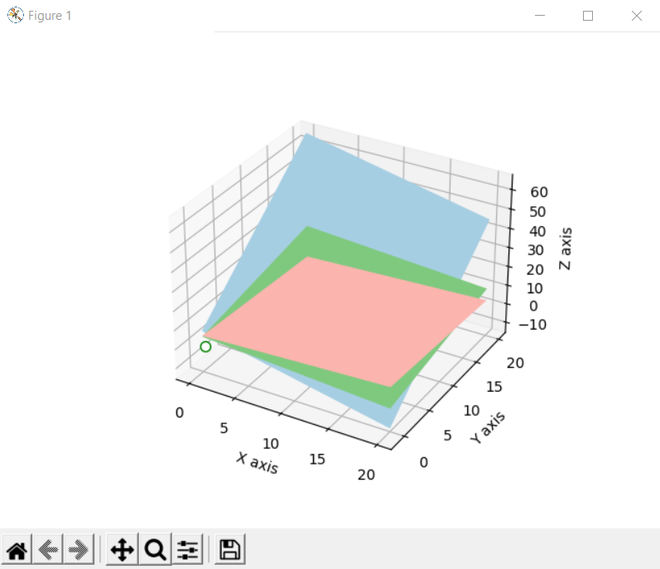# Solve Linear Equation and return 3D Graph in Python

• Last Updated : 27 Jun, 2022

In this article, we will make the 3D graph by solving the linear equations using Python.

## Solve Linear Equation in Python

Here we are going to create a different variable for assigning the value into a linear equation and then calculate the value by using linalg.solve() methods.

## Python3

 `# Python program to solve linear``# equation and return 3-d graph`` ` `# IMport the libraries``import` `numpy as np`` ` `x1, y1, z1, w1 ``=` `1``, ``-``2``, ``3``, ``9`` ` `# Take the input for equation-2``x2, y2, z2, w2 ``=` `-``1``, ``3``, ``-``1``, ``-``6`` ` `# Take the input for equation-3``x3, y3, z3, w3 ``=` `2``, ``-``5``, ``5``, ``17`` ` `# Create an array for LHS variables``LHS ``=` `np.array([[x1, y1, z1], ``                ``[x2, y2, z2], ``                ``[x3, y3, z3]])`` ` `# Create another array for RHS variables``RHS ``=` `np.array([w1, w2, w3])`` ` `# Apply linear algebra on any numpy``# array created and printing the output``sol ``=` `np.linalg.solve(LHS, RHS)``print``(sol)`

Output:

`[ 1. -1.  2.]`

## Solve Linear Equation and return 3D Graph

After getting the linear equation we will plot the 3d graph using matplotlib. we create the 3D graphics using figure() function. Moreover, we use add_subplot() method figure module of matplotlib library for adding axes to the figure as part of a subplot arrangement.

## Python3

 `import` `matplotlib.pyplot as plt``from` `matplotlib ``import` `cm`` ` `# Returns number spaces evenly w.r.t``# interval``x_axis, y_axis ``=` `np.linspace(``0``, ``20``, ``10``), ``np.linspace(``0``, ``20``, ``10``)`` ` `# Create a rectangular grid out of``# two given one-dimensional arrays``X, Y ``=` `np.meshgrid(x_axis, y_axis)`` ` `# Make a rectangular grid``# 3-dimensional by calculating z1, z2, z3``Z1 ``=` `(w1``-``x1``*``X``-``y1``*``Y)``/``z1``Z2 ``=` `(w2``-``x2``*``X``-``y2``*``Y)``/``z2``Z3 ``=` `(w3``+``X``-``Y)``/``z3`` ` `# Create 3D graphics and add``# an add an axes to the figure``fig ``=` `plt.figure()``ax ``=` `fig.add_subplot(``111``, projection``=``'3d'``)`` ` `# Create a 3D Surface Plot``ax.plot_surface(X, Y, Z1, alpha``=``1``, ``                ``cmap``=``cm.Accent, ``                ``rstride``=``100``, cstride``=``100``)``ax.plot_surface(X, Y, Z2, alpha``=``1``, ``                ``cmap``=``cm.Paired, ``                ``rstride``=``100``, cstride``=``100``)``ax.plot_surface(X, Y, Z3, alpha``=``1``,``                ``cmap``=``cm.Pastel1, ``                ``rstride``=``100``, cstride``=``100``)`` ` `# Draw points and make lines``ax.plot((sol[``0``],), (sol[``1``],), (sol[``2``],),``        ``lw``=``2``, c``=``'k'``, marker``=``'o'``,``        ``markersize``=``7``, markeredgecolor``=``'g'``,``        ``markerfacecolor``=``'white'``)`` ` `# Set the label for x-axis, y-axis and``# z-axis``ax.set_xlabel(``'X axis'``)``ax.set_ylabel(``'Y axis'``)``ax.set_zlabel(``'Z axis'``)`` ` `# Display all figures``plt.show()`

Output:My Personal Notes arrow_drop_up